# Numbers up to 8-Digits

Go back to  'Number Systems'

 1 Introduction to Numbers up to 8-Digits 2 The Big Idea: Numbers up to 8-Digits 3 How to Read 8-Digit Numbers? 4 How to Decompose 8-Digit Numbers? 5 How is it important? 6 Important Notes 7 Solved Examples 8 Challenging Questions 9 Practice Questions 10 Frequently Asked Questions (FAQs)

We at Cuemath believe that Math is a life skill. Our Math Experts focus on the “Why” behind the “What.” Students can explore from a huge range of interactive worksheets, visuals, simulations, practice tests, and more to understand a concept in depth.

Book a FREE trial class today! and experience Cuemath's LIVE Online Class with your child.

## Introduction to Numbers up to 8-Digits

Almost 3 centuries ago, when modern society first started to count numbers, the count was done by a system of tallying.

So 4 straight marks were followed by a fifth one placed across the four made a count of five.

Such sets of 5 were used to count up to 10, then 50, 100, 1000 and more.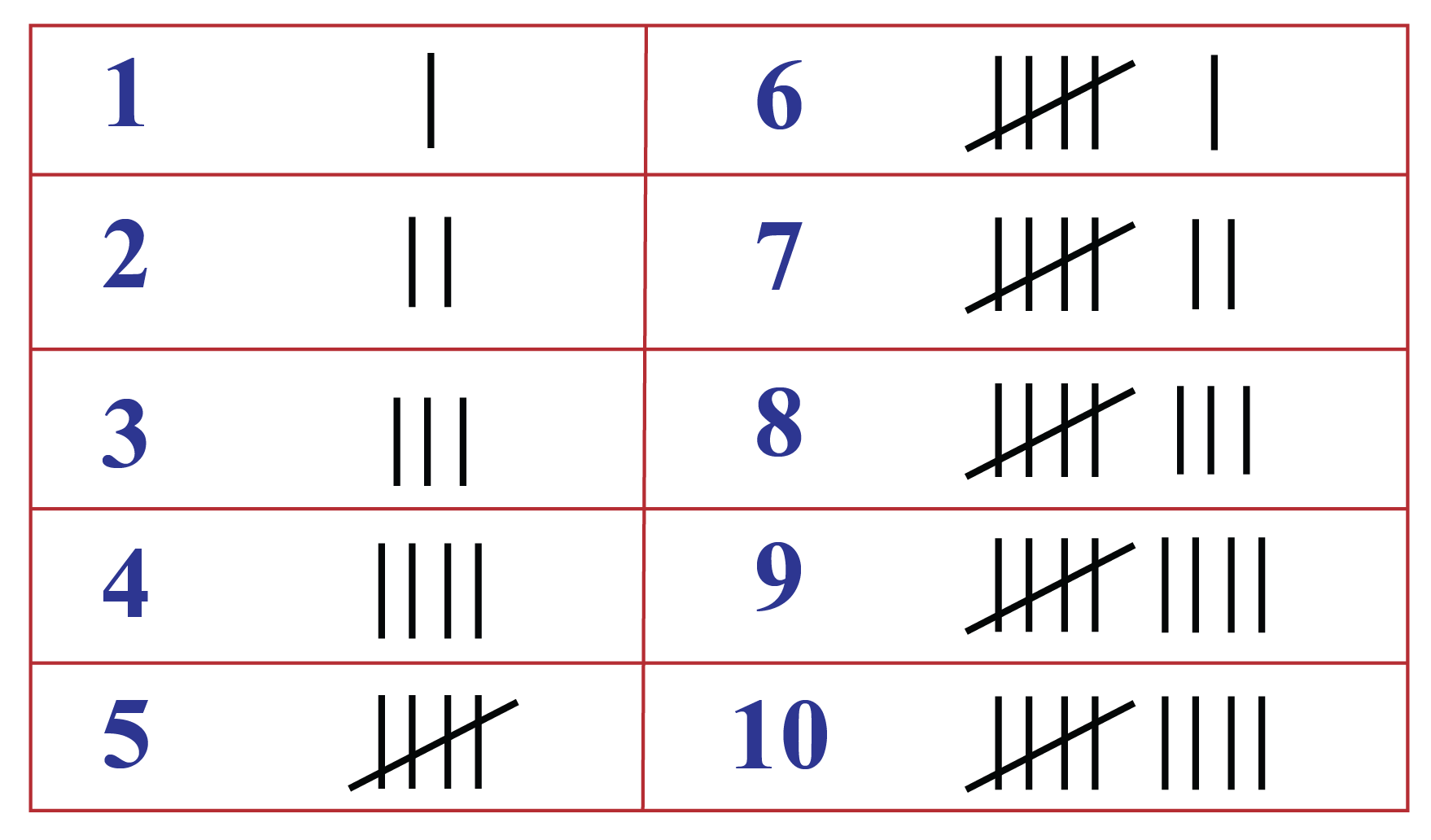In England, there were actually wooden tally sticks made for the purpose of counting larger numbers.

These tally sticks were stored in the House of Commons. They were finally abolished in 1826 and were ordered to be burnt.

But the burning took place inside the House, and by mistake the furnishings caught fire.

As a result, the House burnt down completely.

This was depicted in a famous painting by Turner in 1835

Imagine the number of tally sticks that would have been needed to count larger numbers like lakhs and millions.

Today we go up one more level in our journey towards large numbers, and we will talk about 8 digit numbers today.

## The Big Idea: Numbers up to 8-Digits

### A Simple Idea: The Introduction of a Crore

As we moved from 6 digits to 7 digits, we got acquainted with a new word – million.

This word is used in the International numbering system and is equivalent to 10 lakhs, where a lakh is a word used in the Indian numbering system and is equal to a hundred thousand.When we move on to 8 digits, we get another new word, but this time in the Indian system of numbering.

The smallest 8 digit number is 1 followed by 7 zeros and is written as 10000000

If we write this number in both ways, this is how they would look:

Indian Number System – 1,00,00,000
International Number System – 10,000,000

As per the International system, the placement of the commas can easily tell you that the number is 10 million because the comma after the sixth digit from right signifies 1 million.

In the Indian system, you will see that there is 100 on the left of the comma following the first 5 digits from the right.

So this number is 100 lakhs.

We have a word for 100 lakhs, and it is called a crore.

So as per the Indian numbering system the smallest 8 digit number is a crore, which is equal to a hundred lakhs, or ten million.

## How to Read 8-Digit Numbers?

### The two ways of looking at 8-digit numbers

Eight digit numbers can be expressed in both the Indian systems of numbers and the International system of numbers.

Let us take the random eight digit number 24864701.

Let us look at the two ways we can write this number:

Indian: 2,48,64,701 (this can be read as two crores forty-eight lakhs sixty-four thousand seven hundred and one)

International: 24,864,701 (this can be written as twenty-four million, eight hundred sixty-four thousand seven hundred and one)

## How to Decompose 8-Digit Numbers?

Any eight digit number has place values up to one crore (or ten million).

These are the names of the place values (starting from the right) in an eight digit number:

• Digit 1 – Units
• Digit 2 – Tens
• Digit 3 – Hundreds
• Digit 4 – Thousands
• Digit 5 – Ten Thousands
• Digit 6 – Lakhs
• Digit 7 – Ten Lakhs / Millions
• Digit 8 – Crores / Ten Millions

So let us take the seven digit number we discussed above - 24864701, and see how it gets decomposed.

Here, PV stands for Place Value

 Digit 1 PV = 1 x 1               = 1 Digit 2 PV = 0 x 10             = 00 Digit 3 PV = 7 x 100           = 700 Digit 4 PV = 4 x 1000         = 4000 Digit 5 PV = 6 x 10000       = 60000 Digit 6 PV = 8 x 100000     = 800000 Digit 7 PV = 4 x 1000000   = 4000000 Digit 8 PV = 2 x 10000000 = 20000000

## How is it Important?

### The Zeros in 8-Digit Numbers

There are 8 place values in every 8 digit number, and any number from 0 to 9 can be put into any of these place values.

There is only one exception.

If the leftmost place value (Ten Millions / Crores) is zero, then it becomes a 7-digit number.

If the digits in 24864701 are rearranged to place the zero on the extreme left, the number becomes 04864721, in which case it becomes the 7-digit number 4864721

Help your child score higher with Cuemath’s proprietary FREE Diagnostic Test. Get access to detailed reports, customized learning plans, and a FREE counseling session. Attempt the test now.

Try this simulation to see how the commas are placed in any number according to the Indian Place Value System and the International System.

You can check for numbers up to 10 digits.

Click on RESET, Enter the number and then click GO!

## Solved Examples

 Example 1

Write this 8-digit number in the expanded form (Indian System): 8,53,48,732

Solution:

8,53,48,732 can be expanded in three forms:

8,53,48,732 = 8 crore + 5 ten lakhs + 3 lakhs + 4 ten thousands + 8 thousands + 7 hundreds + 3 tens + 2 ones

8,53,48,732 = $$(8\times 10000000)$$ +  $$(5\times 1000000)$$ +  $$(3\times 100000)$$ +  $$(4\times 10000)$$ +  $$(8\times 1000)$$ +  $$(7\times 100)$$+  $$(3 \times 10)$$ +  $$(2\times 1)$$

$$8,53,48,732$$ = $$8,00,00,000$$ + $$50,00,000$$ + $$3,00,000$$ + $$40,000$$ + $$8,000$$ + $$700$$ + $$30$$ + $$2$$

 Example 2

Write the predecessor and successor of 6,75,42,099 and their number names according to the Indian Place value system.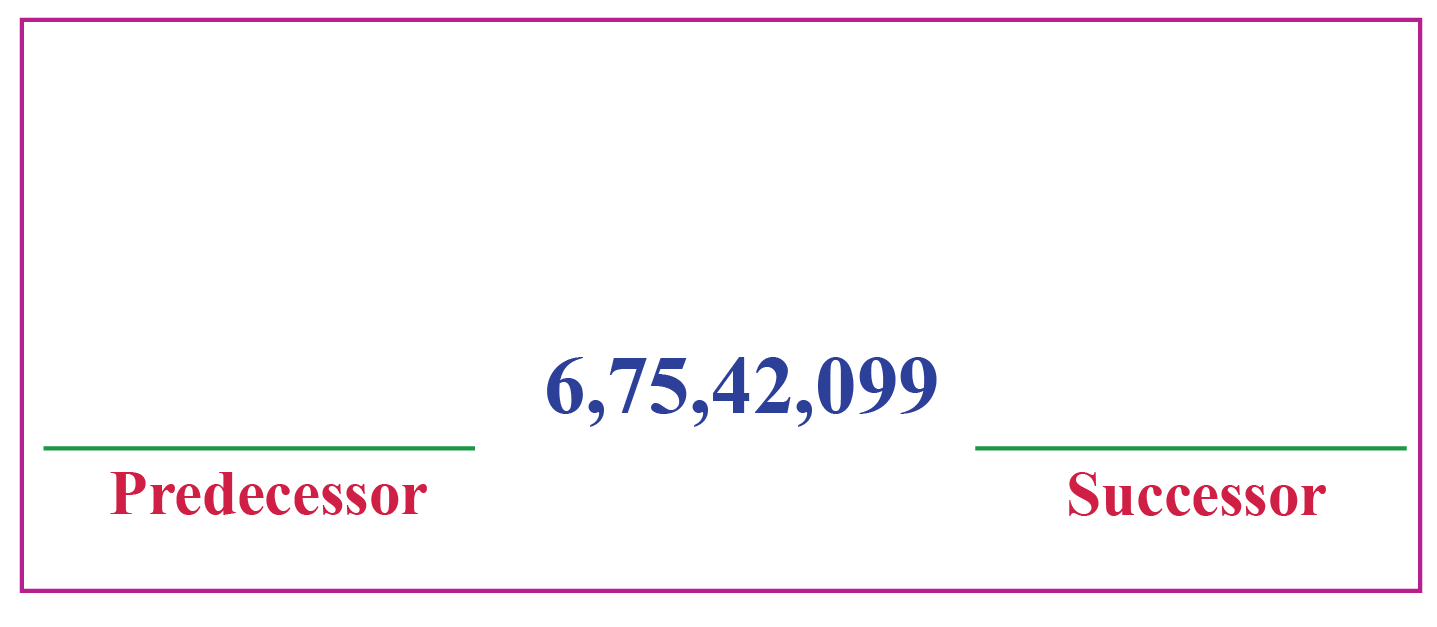Solution:

The predecessor of 6,75,42,099 is 6,75,42,098

Number name: Six crore, seventy-five lakh, forty-two thousand and ninety-eight

The successor of 6,75,42,099 is 6,75,42,100

Number name: Six crore, seventy-five lakh, forty-two thousand and one hundred

 Example 3

There are 8 blocks with numbers printed on them.

Arrange them to find the greatest 8-digit number which can be formed with no digit repeated.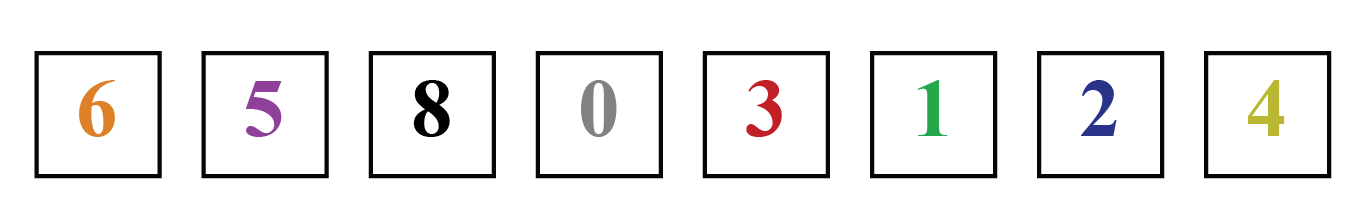Solution:

For the greatest number, the digit on the Crore place will be the greatest: 8

Now the other numbers will be arranged in the descending order: 8,65,43,210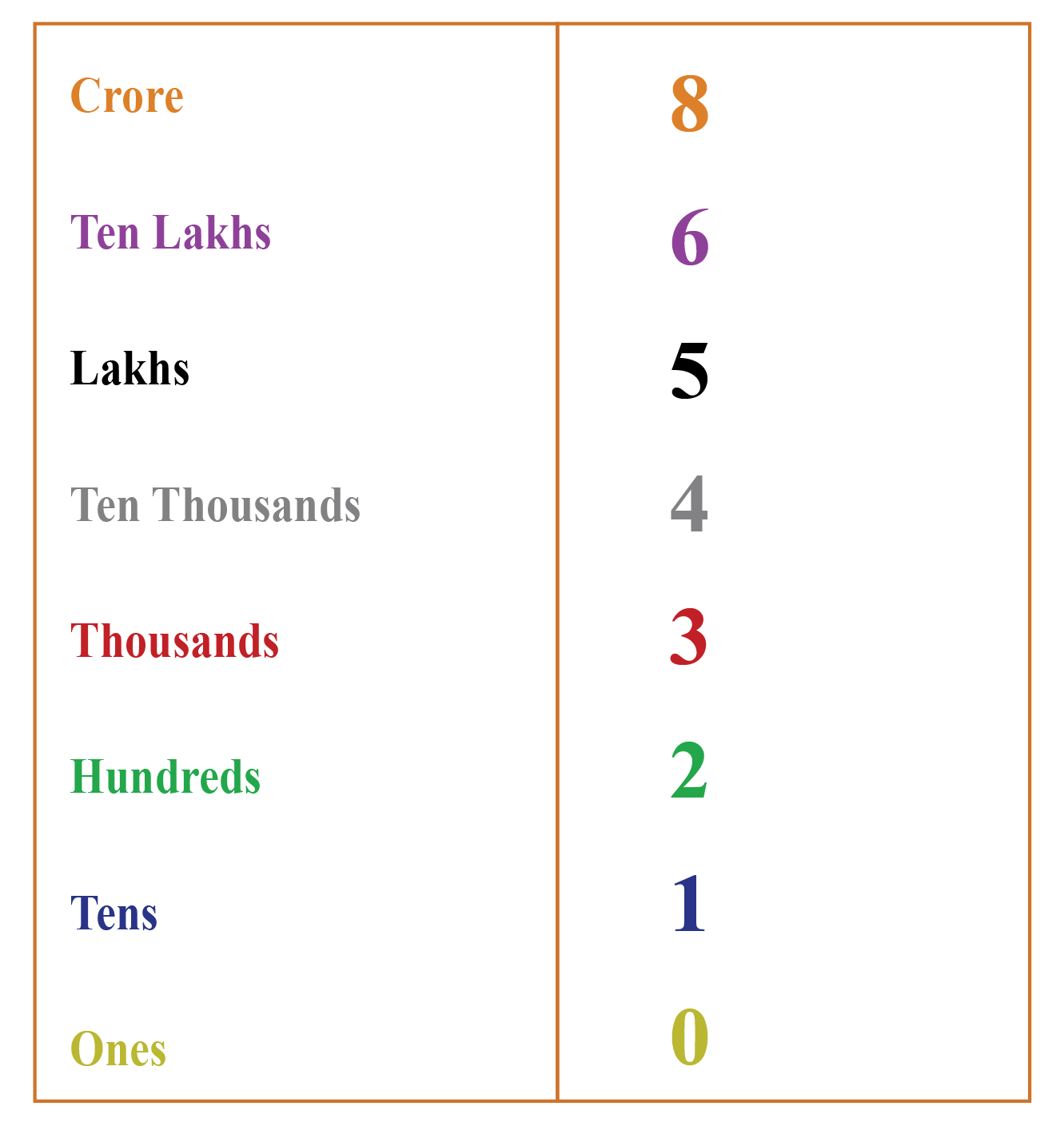The number is 8,65,43,210
 Example 4

Write the 4 consecutive numbers after 7,34,14,198 along with their number names according to the Indian Place Value System.

Solution:

The 4 consecutive numbers which come after 7,34,14,198 are:

7,34,14,199 - Seven Crore, thirty-four lakh, fourteen thousand, one hundred and ninety nine

7,34,14,200 - Seven Crore, thirty-four lakh, fourteen thousand and two hundred

7,34,14,201 - Seven Crore, thirty-four lakh, fourteen thousand, two hundred and one

7,34,14,202 - Seven Crore, thirty-four lakh, fourteen thousand, two hundred and two

 Example 5

Find the difference between the greatest 8-digit number and the smallest 6-digit number.

Write the predecessor and successor of the result obtained.

Solution:

The greatest 8-digit number is 9,99,99,999

The smallest 6-digit number is 1,00,000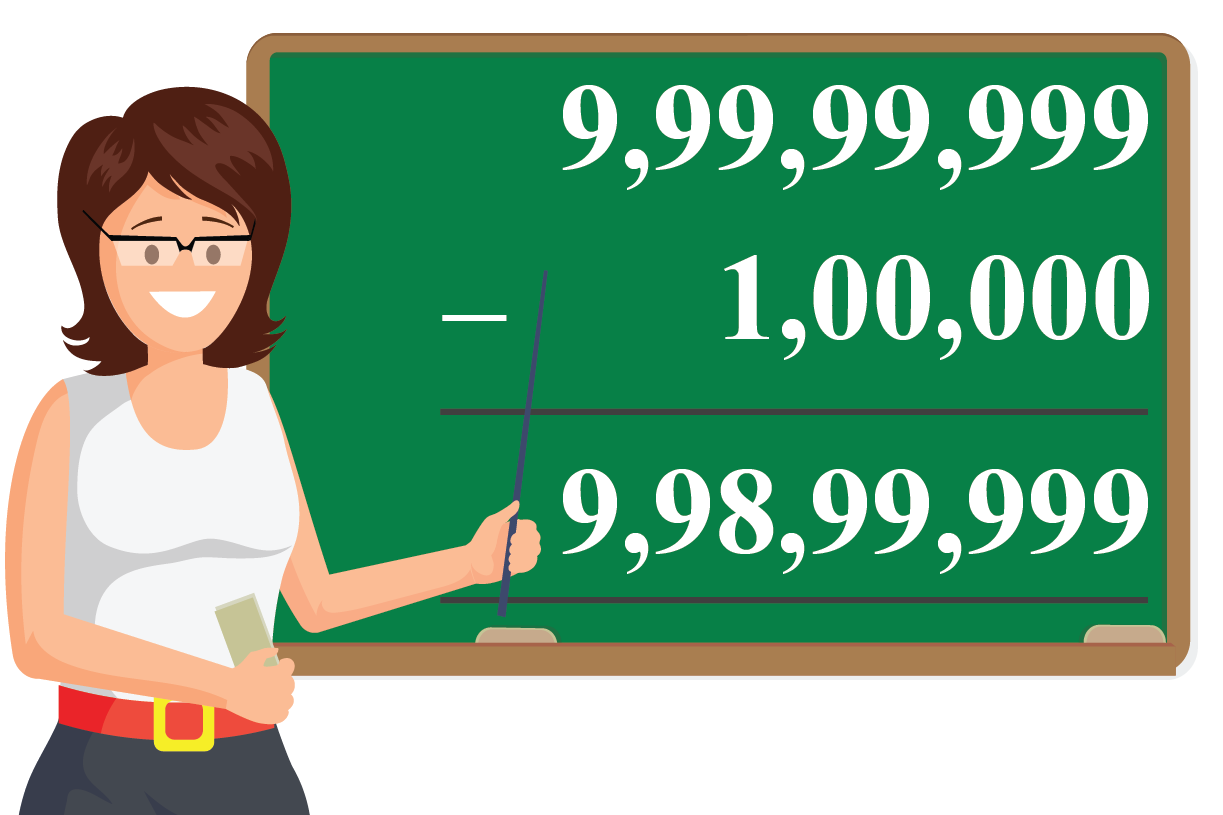Their difference is 9,99,99,999 - 1,00,000 = 9,98,99,999

The predecessor of 9,98,99,999  is 9,98,99,998

The successor of 9,98,99,999 is 9,99,00,000Challenging Questions
1. How many hundreds make one crore?
2. Arrange the following numbers in ascending order: 3,22,33,232; 3,33,22,323; 3,32,23,222; 3,22,33,333

Have a doubt that you want to clear? Get it clarified with simple solutions on Numbers up to 8-digits from our Math Experts at Cuemath’s LIVE, Personalised and Interactive Online Classes.

Make your kid a Math Expert, Book a FREE trial class today!

## Practice Questions

Here are a few activities for you to practice. Select/Type your answer and click the "Check Answer" button to see the result.

## 1. What is the largest 8 digit number?

The greatest 8-digit number is 9,99,99,999 which is read as Nine crore, ninety-nine lakh, ninety-nine thousand, nine hundred and ninety-nine.

## 2. How many unique numbers are there in 8 digits?

Unique numbers in 8-digits indicates the total number of 8-digit numbers.

There are nine crore (9,00,00,000) 8-digit numbers in all.

## 3. What is the smallest 8 digit number?

The smallest 8-digit number is 1,00,00,000 which is read as One crore.

## 4. What is the greatest 8 digit number having three different digits?

The greatest 8-digit number having three different digits is 9,99,99,987, which is read as Nine crore, ninety-nine lakh, ninety-nine thousand, nine hundred and eighty seven.

More Important Topics
Numbers
Algebra
Geometry
Measurement
Money
Data
Trigonometry
Calculus